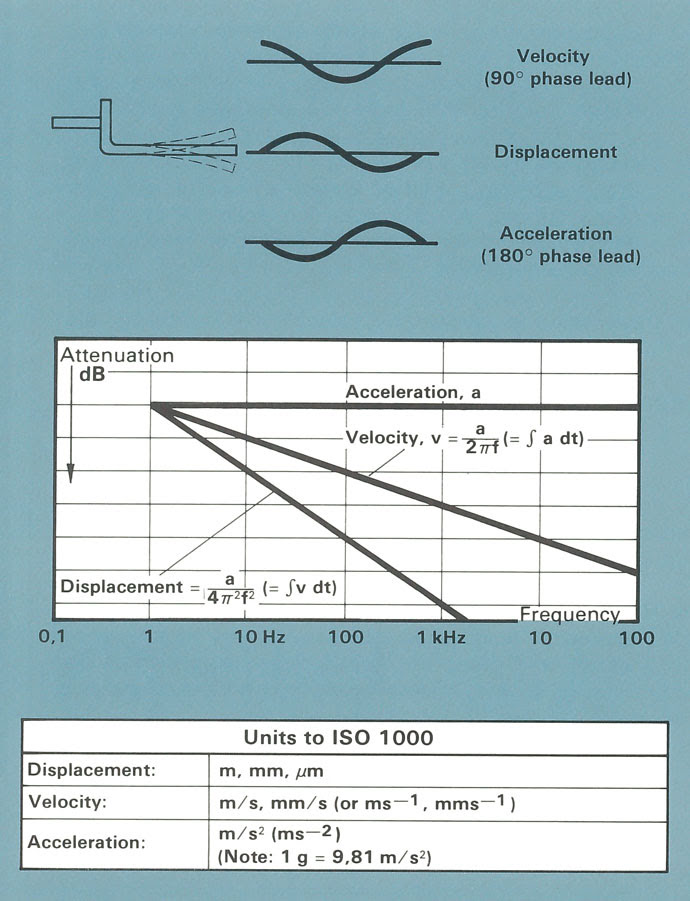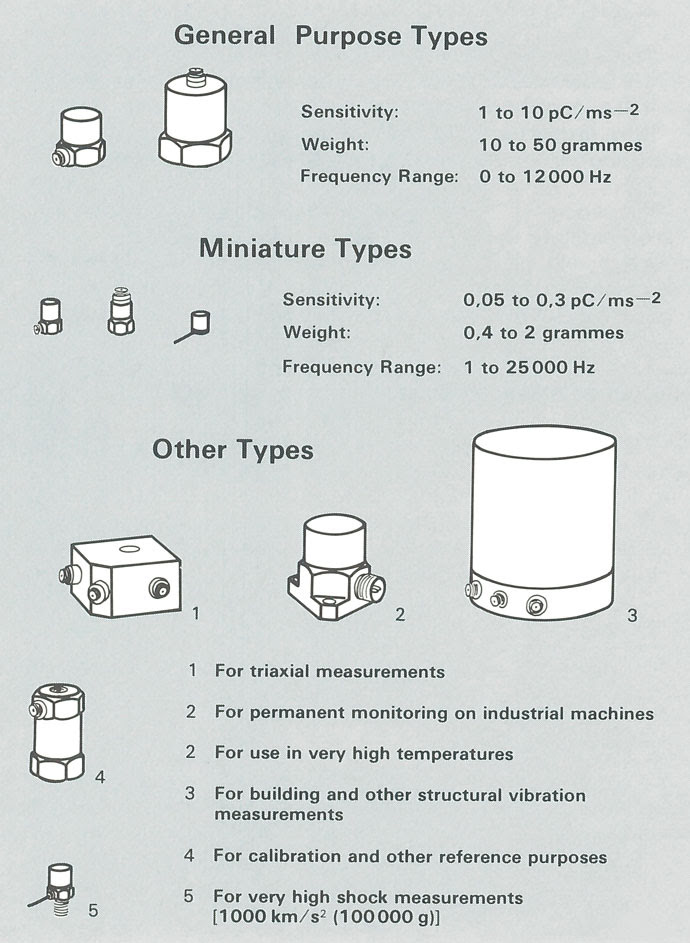# Measuring Vibration

Over the last 15 or 20 years a whole new technology of vibration measurement has been developed which is suitable for investigating modern highly stressed, high speed machinery. Using piezoelectric accelerometers to convert vibratory motion into an electrical signal, the process of measurement and analysis is ably performed by the versatile abilities of electronics.

## What is Vibration?

A body is said to vibrate when it describes an oscillating motion about a reference position. The number of times a complete motion cycle takes place during the period of one second is called the Frequency and is measured in hertz (Hz).

The motion can consists of a single component occurring at a single frequency, as with a tuning fork, or of several components occurring at different frequencies simultaneously, as for example, with the piston motion of an internal combustion engine.

Vibration signals in practice usually consist of very many frequencies occurring simultaneously so that we cannot immediately see just by looking at the amplitude-time pattern, how many components there are, and at what frequencies they occur.

These components can be revealed by plotting vibration amplitude against frequency. The breaking down of vibration signals into individual frequency components is called frequency analysis, a technique which may be considered the cornerstone of diagnostic vibration measurements. The graph showing the vibration level as a function of frequency is called a frequency spectrogram.

When frequency analyzing machine vibrations we normally find a number of prominent periodic frequency components which are directly related to the fundamental movements of various parts of the machine. With frequency analysis we are therefore able to track down the source of undesirable vibration.

## Vibration Parameters: Acceleration, Velocity and Displacement.When we looked at the vibrating tuning fork, we considered the amplitude of the wave as the physical displacement of the fork ends to either side of the rest position. In addition to Displacement we can also describe the movement of the fork leg in terms of its velocity and its acceleration. The form and period of the vibration remain the same whether it is the displacement, velocity or acceleration that is being considered. The main difference is that there is a phase difference between the amplitude-time curves of the three parameters as shown in the drawing.

For sinusoidal signals, displacement, velocity and acceleration amplitudes are related mathematically by a function of frequency and time, this is shown graphically in the diagram. If phase is neglected, as is always the case when making time-average measurements, then the velocity level can be obtained by dividing the acceleration signal by a factor proportional to frequency, and the dis- placement can be obtained by dividing the acceleration signal by a factor proportional to the square of frequency. This division is performed by electronic integra- tors in the measuring instrumentation.

The vibration parameters are almost universally measured in metric units in accordance with ISO requirements, these are shown in the table. The gravitational constant "g" is still widely used for acceleration levels al- though it is outside the ISO system of coherent units. Fortunately, a factor of almost 10 (9,81) relates the two units so that mental conversion within 2% is a simple matter.

### Accelerometer TypesMost manufacturers have a wide range of accelerometers, at first sight may be too many to make the choice easy. A small group of "general purpose" types will satisfy most needs. These are available with either top or side mounted connectors and have sensitivities in the range 1 to 10 mV or pC per m/s2. The Brüel & Kjær Uni-Gain® types have their sensitivity normalized to a convenient "round figure" such as 1 or 10 pC/ms-2 to simplify calibration of the measuring system.

The remaining accelerometers have their characteristics slanted towards a particular application. For example, small size acclerometers that are intended for high level or high frequency measurements and for use on delicate structures, panels, etc. and which weigh only 0,5 to 2 grammes.

Other special purpose types are optimized for: simultaneous measurement in three mutually perpendicular planes; high temperatures; very low vibration levels; high level shocks; calibration of other accelerometers by comparison; and for permanent monitoring on industrial machines.

Related Articles